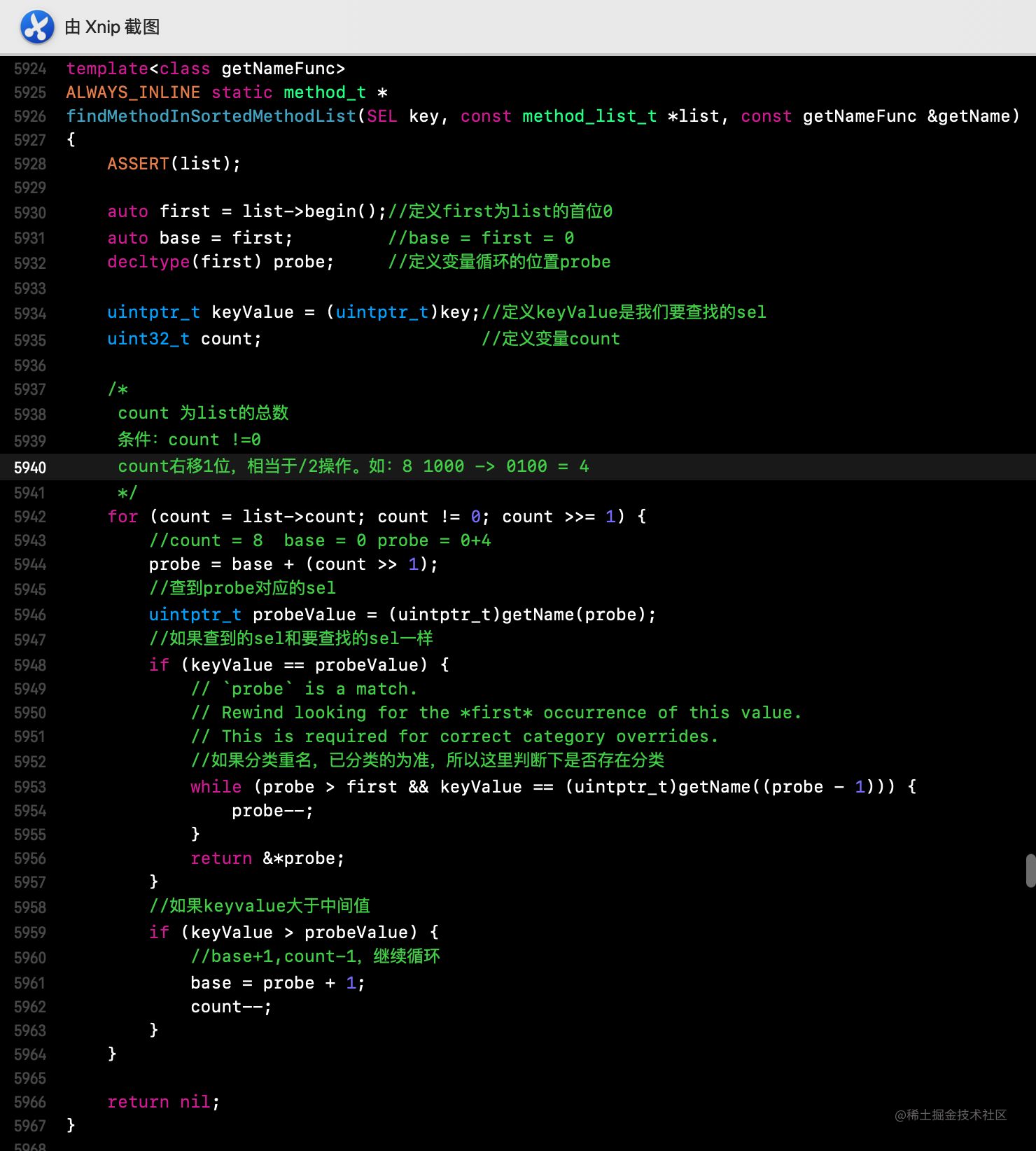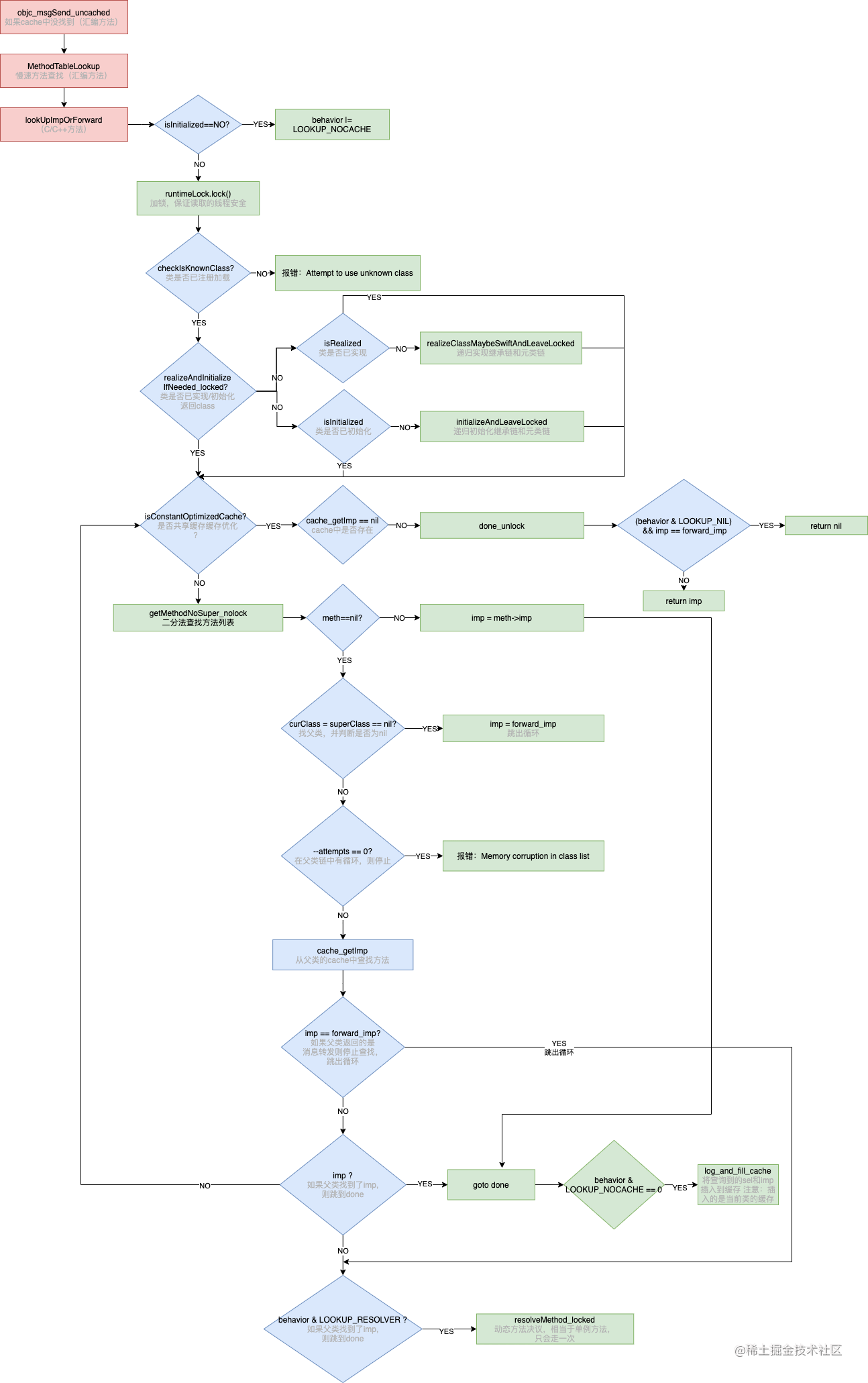# iOS底层原理--方法的慢速查找流程

## 前言

iOS底层原理--RunTime之objc_msgSend探究(快速查找)这篇文章中，我们已经分析了消息的快速查找流程，如果在快速查找流程中没有找到，就会进入到`__objc_msgSend_uncached`，也就是慢速查找流程。下面👇我们就进入到慢速查找流程就行分析。

## 源码分析

``````IMP lookUpImpOrForward(id inst, SEL sel, Class cls, int behavior)
{
/*
定义的消息转发
behavior==3 = LOOKUP_INITIALIZE|LOOKUP_RESOLVER
*/
const IMP forward_imp = (IMP)_objc_msgForward_impcache;
IMP imp = nil;
Class curClass;

runtimeLock.assertUnlocked();

/*
判断类是否已经初始化，如果没有初始化的话
behavior = LOOKUP_NOCACHE|LOOKUP_INITIALIZE|LOOKUP_RESOLVER
*/
if (slowpath(!cls->isInitialized())) {
behavior |= LOOKUP_NOCACHE;
}
//加锁，目的是保证读取的线程安全
runtimeLock.lock();
//是否是已知的类，也就是说该类是否已经加载过了
checkIsKnownClass(cls);
//判断类是否实现，如果没有，需要先实现，此时的目的是为了确定父类链，方法后续的循环
cls = realizeAndInitializeIfNeeded_locked(inst, cls, behavior & LOOKUP_INITIALIZE);
// runtimeLock may have been dropped but is now locked again
runtimeLock.assertLocked();
curClass = cls;
//死循环
for (unsigned attempts = unreasonableClassCount();;) {
////判断是否有共享缓存缓存优化
if (curClass->cache.isConstantOptimizedCache(/* strict */true)) {
#if CONFIG_USE_PREOPT_CACHES
/*
如果是真机，再走一次快速查找流程，
可能在走的这步的过程中，别的线程调用了该方法，严谨一些
*/
imp = cache_getImp(curClass, sel);
//如果缓存中找到imp，则跳转到done_unlock
if (imp) goto done_unlock;
curClass = curClass->cache.preoptFallbackClass();
#endif
} else {
// curClass method list.
//采用二分法查找methodList
Method meth = getMethodNoSuper_nolock(curClass, sel);
//如果找到了，则获取imp跳到done.
if (meth) {
imp = meth->imp(false);
goto done;
}
//直到当前类的父类为nil为止（也就是NSObject），将定义的消息转发赋值给imp，跳出循环
if (slowpath((curClass = curClass->getSuperclass()) == nil)) {
// No implementation found, and method resolver didn't help.
// Use forwarding.
imp = forward_imp;
break;
}
}

// Halt if there is a cycle in the superclass chain.
//如果在父类链中有循环，则停止
if (slowpath(--attempts == 0)) {
_objc_fatal("Memory corruption in class list.");
}

// Superclass cache.
// 从父类cache中找（快速查找）
imp = cache_getImp(curClass, sel);
//如果父类返回的是forward_imp 停止查找，跳出循环
if (slowpath(imp == forward_imp)) {
// Found a forward:: entry in a superclass.
// Stop searching, but don't cache yet; call method
// resolver for this class first.
break;
}
//如果父类找到了imp,则跳到done
if (fastpath(imp)) {
// Found the method in a superclass. Cache it in this class.
goto done;
}
}

// No implementation found. Try method resolver once.
/*
behavior = 3  LOOKUP_RESOLVER = 2
3&2 = 0011 & 0010 = 0010 与操作全部为1才为1
3^2 = 0011 ^ 0010 = 0001 = behavior
所以第二次进来 1 & 2 = 0001 & 0010 = 0000 条件不成立也就进不来了
*/
if (slowpath(behavior & LOOKUP_RESOLVER)) {
behavior ^= LOOKUP_RESOLVER;
//动态方法决议
return resolveMethod_locked(inst, sel, cls, behavior);
}

done:
// 3 & 8 = 0011 & 1000 = 0000 = 0
if (fastpath((behavior & LOOKUP_NOCACHE) == 0)) {
#if CONFIG_USE_PREOPT_CACHES
while (cls->cache.isConstantOptimizedCache(/* strict */true)) {
cls = cls->cache.preoptFallbackClass();
}
#endif
//将查询到的sel和imp插入到缓存 注意：插入的是当前类的缓存
log_and_fill_cache(cls, imp, sel, inst, curClass);
}
done_unlock:
runtimeLock.unlock();

if (slowpath((behavior & LOOKUP_NIL) && imp == forward_imp)) {
return nil;
}
return imp;
}

### `慢速查找流程总结`

• 定义消息转发IMP
• 判断类是否已经初始化
• 类是否已加载
• 类是否已实现，确定父类/元类链路，方便进行递归查找
• 递归查找
• 判断是否有共享缓存优化
• 如果有，走快速查找流程，找到后，插入缓存返回IMP
• 如果没有，进行二分法在当前类中methodList中找method，如果找到，则返回对应的IMP，如果没有找到，继续执行。直到找到NSObject，跳出循环。
• 如果父类链中有循环，则抛异常停止
• 在父类cache中快速查找IMP
• 如果父类返回的是forward_imp 停止查找，跳出循环
• 如果父类找到了imp,则将查询到的sel和imp插入到缓存 注意：插入的是当前类的缓存
• 如果cls以及父类都没有查询到，此时系统会给你一次机会，判断是否执行过动态方法决议，如果没有则走动态方法决议。

### 各个方法详解

#### initializeAndLeaveLocked

`对象方法: 先从当前类中找，没有接着去父类中找，还没有再接着去爷爷类中找->...->最后到根类中找。`
`类方法: 先从当前类的元类中找，没有去父元类中找->...->没有再去根元类找。`

### 二分查找法

#### findMethodInSortedMethodList

`前提：` `非常重要的一个前提是，列表已经经过排序了，否则无法使用二分法。``举个🌰`

• count = 8, base = 0, probe = 0+4
• 2!=4
• 2<4

• count = 8>>1 = 4; base = 0,probe = 0+2
• 2==2,找到方法，返回

`再举个🌰`

• count = 8, base = 0, probe = 0+4
• 7!=4
• 7>4, base = 4+1 = 5, count = 8-1 = 7

• count = 7>>1 = 3, base = 5, probe = 5+1 = 6
• 7!=6
• 7>6, base = 6+1 = 7, count = 3-1 = 2

• count = 2>>1 = 1, base = 7, probe = 7+0 =7
• 7==7，找到方法，返回

#### 全文总结

• 实例方法（对象方法），先在当前类中进行快速查找。
• 如果在cache中找不到，会进行慢速查找。
• 慢速找到：方法存入cache，返回imp。
• 找不到：依次类--父类--根类--nil，父类只会cache中找，找不到直接返回nil。
• 找到，直接返回IMP。
• 类方法，先在元类中快速查找，其慢速查找的父类链是：元类--根元类--根类--nil
• 如果在cache中找不到，会进行慢速查找。
• 找到：方法存入cache，返回imp。
• 找不到：依次元类--根元类--根类--nil。
• 找到，直接返回IMP。
• 如果快速查找、慢速查找都找不大方法的实现（IMP），则尝试`动态方法决议`
• 如果动态方法决议依然找不到，则会进行`消息转发`

### 慢速查找流程图